# Binary Search Tree | Example | Construction

## Binary Tree-

Before you go through this article, make sure that you gone through the previous article on Binary Trees.

We have discussed-

• Binary tree is a special tree data structure.
• In a binary tree, each node can have at most 2 children.
• In a binary tree, nodes may be arranged in any random order.

## Binary Search Tree-

 Binary Search Tree is a special kind of binary tree in which nodes are arranged in a specific order.

In a binary search tree (BST), each node contains-

• Only smaller values in its left sub tree
• Only larger values in its right sub tree

## Example-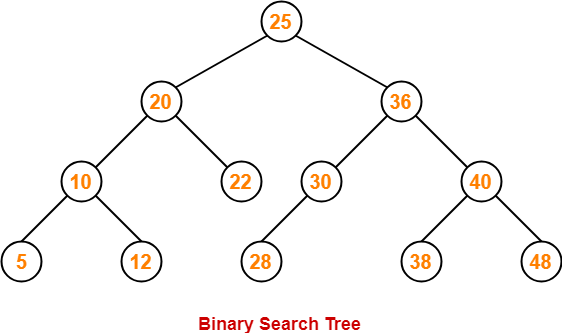## Number of Binary Search Trees-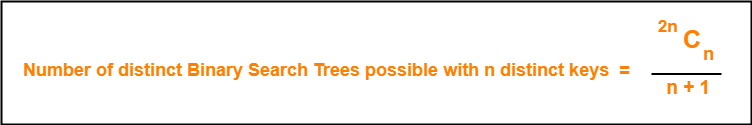## Example-

Number of distinct binary search trees possible with 3 distinct keys

= 2×3C3 / 3+1

= 6C3 / 4

= 5

If three distinct keys are A, B and C, then 5 distinct binary search trees are-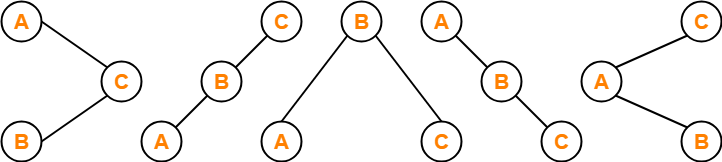## Binary Search Tree Construction-

Let us understand the construction of a binary search tree using the following example-

## Example-

Construct a Binary Search Tree (BST) for the following sequence of numbers-

50, 70, 60, 20, 90, 10, 40, 100

When elements are given in a sequence,

• Always consider the first element as the root node.
• Consider the given elements and insert them in the BST one by one.

The binary search tree will be constructed as explained below-

### Insert 50-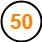### Insert 70-

• As 70 > 50, so insert 70 to the right of 50.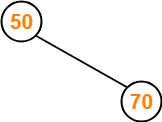### Insert 60-

• As 60 > 50, so insert 60 to the right of 50.
• As 60 < 70, so insert 60 to the left of 70.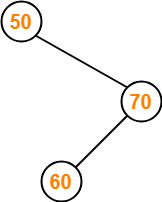### Insert 20-

• As 20 < 50, so insert 20 to the left of 50.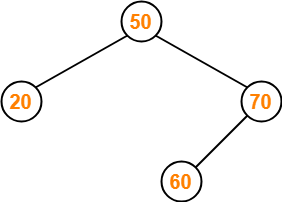### Insert 90-

• As 90 > 50, so insert 90 to the right of 50.
• As 90 > 70, so insert 90 to the right of 70.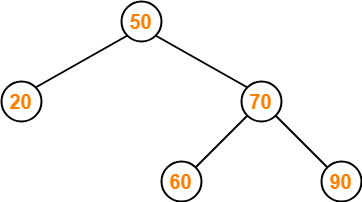### Insert 10-

• As 10 < 50, so insert 10 to the left of 50.
• As 10 < 20, so insert 10 to the left of 20.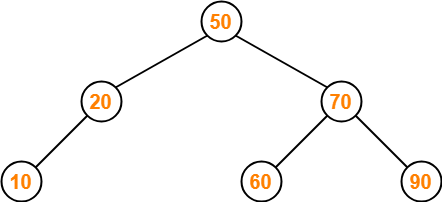### Insert 40-

• As 40 < 50, so insert 40 to the left of 50.
• As 40 > 20, so insert 40 to the right of 20.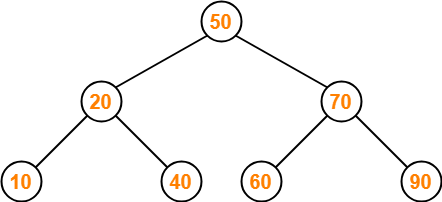### Insert 100-

• As 100 > 50, so insert 100 to the right of 50.
• As 100 > 70, so insert 100 to the right of 70.
• As 100 > 90, so insert 100 to the right of 90.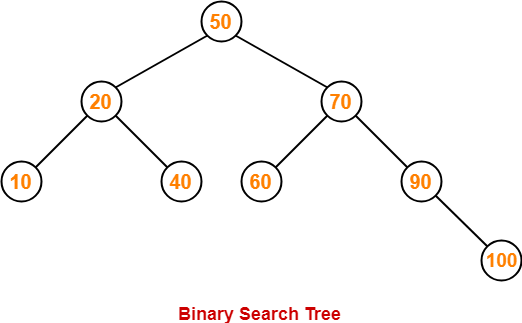This is the required Binary Search Tree.

To gain better understanding about Binary Search Trees,

Watch this Video Lecture

## Problem-01:

A binary search tree is generated by inserting in order of the following integers-

50, 15, 62, 5, 20, 58, 91, 3, 8, 37, 60, 24

The number of nodes in the left subtree and right subtree of the root respectively is _____.

1. (4, 7)
2. (7, 4)
3. (8, 3)
4. (3, 8)

## Solution-

Using the above discussed steps, we will construct the binary search tree.

The resultant binary search tree will be-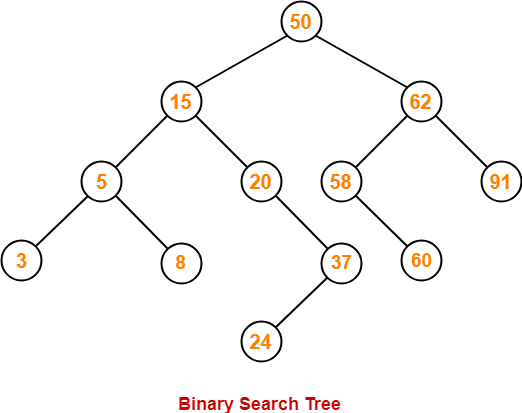Clearly,

• Number of nodes in the left subtree of the root = 7
• Number of nodes in the right subtree of the root = 4

Thus, Option (B) is correct.

## Problem-02:

How many distinct binary search trees can be constructed out of 4 distinct keys?

1. 5
2. 14
3. 24
4. 35

## Solution-

Number of distinct binary search trees possible with 4 distinct keys

= 2nCn / n+1

2×4C4 / 4+1

= 8C4 / 5

= 14

Thus, Option (B) is correct.

## Problem-03:

The numbers 1, 2, …, n are inserted in a binary search tree in some order. In the resulting tree, the right subtree of the root contains p nodes. The first number to be inserted in the tree must be-

1. p
2. p+1
3. n-p
4. n-p+1

## Solution-

Let n = 4 and p = 3.

Then, given options reduce to-

1. 3
2. 4
3. 1
4. 2

Our binary search tree will be as shown-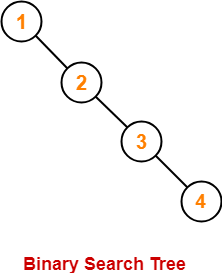Clearly, first inserted number = 1.

Thus, Option (C) is correct.

## Problem-04:

We are given a set of n distinct elements and an unlabeled binary tree with n nodes. In how many ways can we populate the tree with given set so that it becomes a binary search tree?

1. 0
2. 1
3. n!
4. C(2n, n) / n+1

## Solution-

Option (B) is correct.

To watch video solutions and practice more problems,

Watch this Video LectureNext Article- Binary Search Tree Traversal

Get more notes and other study material of Data Structures.

Watch video lectures by visiting our YouTube channel LearnVidFun.

SummaryArticle Name
Binary Search Tree | Example | Construction
Description
Binary Search Tree or BST is a special kind of binary tree. Binary Search Tree Example is given. Binary Search Tree Construction- Various steps to construct binary search tree are given. Practice Problems on Binary Search Trees.
Author
Publisher Name
Gate Vidyalay
Publisher Logo